# Mechanics LecturePage 3

#### WATCH ALL SLIDES

If an object that can be modeled as a particle experiences an acceleration, there must be a nonzero net force acting on it

Draw a free-body diagram

Apply Newton’s Second Law in component form

Slide 16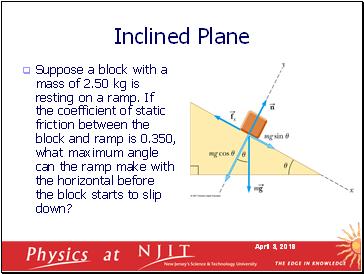## Inclined Plane

Suppose a block with a mass of 2.50 kg is resting on a ramp. If the coefficient of static friction between the block and ramp is 0.350, what maximum angle can the ramp make with the horizontal before the block starts to slip down?

Slide 17Newton 2nd law:

Then

So

Inclined Plane

Slide 18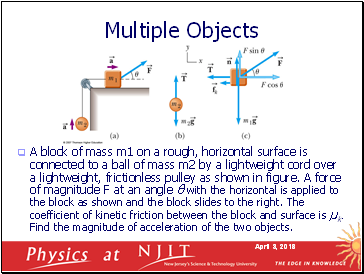## Multiple Objects

A block of mass m1 on a rough, horizontal surface is connected to a ball of mass m2 by a lightweight cord over a lightweight, frictionless pulley as shown in figure. A force of magnitude F at an angle θ with the horizontal is applied to the block as shown and the block slides to the right. The coefficient of kinetic friction between the block and surface is μk. Find the magnitude of acceleration of the two objects.

Slide 19Multiple Objects

m1:

m2:

Slide 20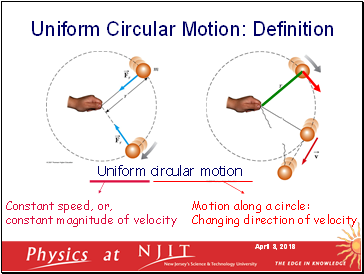Uniform circular motion

Constant speed, or,

constant magnitude of velocity

Motion along a circle:

Changing direction of velocity

Uniform Circular Motion: Definition

Slide 21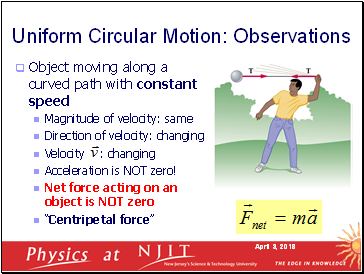## Uniform Circular Motion: Observations

Object moving along a curved path with constant speed

Magnitude of velocity: same

Direction of velocity: changing

Velocity : changing

Acceleration is NOT zero!

Net force acting on an object is NOT zero

“Centripetal force”

Slide 22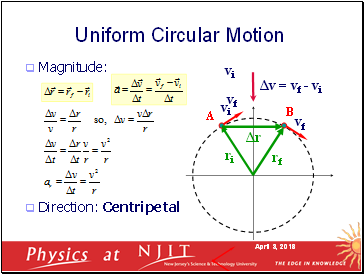Magnitude:

Direction: Centripetal

Uniform Circular Motion

O

x

y

ri

R

A

B

vi

rf

vf

Δr

vi

vf

Δv = vf - vi

Slide 23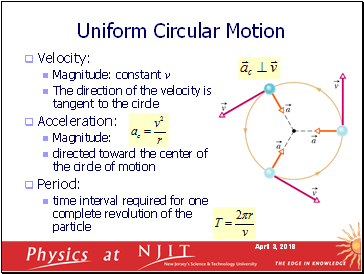## Uniform Circular Motion

Velocity:

Magnitude: constant v

The direction of the velocity is tangent to the circle

Acceleration:

Magnitude:

directed toward the center of the circle of motion

Period:

time interval required for one complete revolution of the particle

Slide 24Centripetal Force

Acceleration:

Magnitude:

Direction: toward the center of the circle of motion

Go to page:
1  2  3  4  5